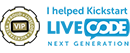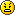## Converting Excel formula into LC

Want to move your code and projects to LiveCode but don't know where to start?

Moderators: Klaus, FourthWorld, heatherlaine, robinmiller

Simon
VIP Livecode Opensource BackerPosts: 3901
Joined: Sat Mar 24, 2007 2:54 am
Location: Palo Alto

### Converting Excel formula into LC

Hello,
I'm trying to change this code:

Code: Select all

``MOD(DEGREES(ACOS(((SIN(RADIANS(B3))*COS(RADIANS(AD2)))-SIN(RADIANS(T2)))/(COS(RADIANS(B3))*SIN(RADIANS(AD2)))))+180,360)``
Into an LC function.

When I thought I had it right the results wouldn't match up to Excels results.Any help welcome.
Thank you,
Simon
I used to be a newbie but then I learned how to spell teh correctly and now I'm a noob!

Simon
VIP Livecode Opensource BackerPosts: 3901
Joined: Sat Mar 24, 2007 2:54 am
Location: Palo Alto

### Re: Converting Excel formula into LC

Just thought I'd bump this message.

Thanks,
Simon
I used to be a newbie but then I learned how to spell teh correctly and now I'm a noob!

Simon
VIP Livecode Opensource BackerPosts: 3901
Joined: Sat Mar 24, 2007 2:54 am
Location: Palo Alto

### Re: Converting Excel formula into LC

Got It!Code: Select all

``````Excel
MOD(DEGREES(ACOS(((SIN(RADIANS(B3))*COS(RADIANS(AD2)))-SIN(RADIANS(T2)))/(COS(RADIANS(B3))*SIN(RADIANS(AD2)))))+180,360)
In LC becomes
((ACOS(((SIN((B3)*pi/180)*COS((AD2)*pi/180))-SIN((T2)*pi/180))/(COS((B3)*pi/180)*SIN((AD2)*pi/180))))*180/pi +180 MOD 360)``````
Too much over thinking, it really was almost a cut and paste.

SimonI used to be a newbie but then I learned how to spell teh correctly and now I'm a noob!